# 3D shapes

Here you will learn about 3D shapes, including their names and properties and how to identify and classify 3D shapes.

Students will first learn about 3D shapes in 1 st grade with their work in geometry, identifying and classifying shapes by their properties. They expand their knowledge of 3D shapes as they progress through elementary and middle school.

## What are 3D shapes?

3D shapes (three-dimensional shapes) are solid shapes that have 3 dimensions: height, width and depth (all line segments).

Here are some familiar 3D shapes:

CubeConePyramid
shape
Cylinder

### Faces, edges and vertices

Polyhedra are 3D shapes made up of faces, edges and vertices as illustrated below.

Face

A flat 2D shape
surrounded by
edges and vertices.

Edge

A straight line
where two faces
meet and connect
two vertices

Vertex

A point where
two or more edges
meet (like a corner)

The shape in the table is a cube. It has 6 faces, 12 edges and 8 vertices.

### Properties of 3D Shapes

3D shapes can be categorized in several ways. A 3D shape is either a polyhedra or a non-polyhedra.

3D shapes can then be further categorized as shown in the table below. The properties of 3D shapes can help to classify them and recall their names.

Name of polyhedraProperties of polyhedra

Rectangular prism

A rectangular prism can be
described as a cuboid.

Step-by-step guide:

Rectangular prism

6 faces
12 edges
8 vertices (corners)

Triangular prism

Step-by-step guide:

Triangular prism

5 faces
9 edges
6 vertices (corners)

Square pyramid

Step-by-step guide:

Square Pyramid

5 faces
8 edges
5 vertices (corners)

Triangular pyramid
(tetrahedron)

Step-by-step guide:

Triangular Pyramid

4 faces
6 edges
4 vertices (corners)

Name of non-polyhedraProperties of non-polyhedra

Sphere

A sphere can be described
simply as a ball.

Step-by-step guide:

Sphere shape

● All dimensions (length, width
and height) are the same.
● The center point is equal from
every point on its surface.

Cylinder

A cylinder can be described as
‘prism-like’ with a circular base.

Step-by-step guide:

Cylinder

2 circular bases
1 curved lateral surface

Cone

A cone can be described as
‘pyramid-like’ with a circular base.

Step-by-step guide:

Cone

1 circular base
1 curved surface
1 apex (vertex)

Step-by-step guide: 3D shape names

### Volume and surface area of 3D shapes

You can find the volume and surface area of 3D shapes.

The volume of a 3D shape is the amount of space there is inside the shape.

Step-by-step guide: Volume

Surface area is the total area occupied by the surface of a 3D shape.

To find the surface area of a prism, cylinder, or pyramid, it is often helpful to draw the net of the 3D shape, calculate the area of each face, and then add them together.

Step-by-step guide: Surface area

### What are 3D shapes?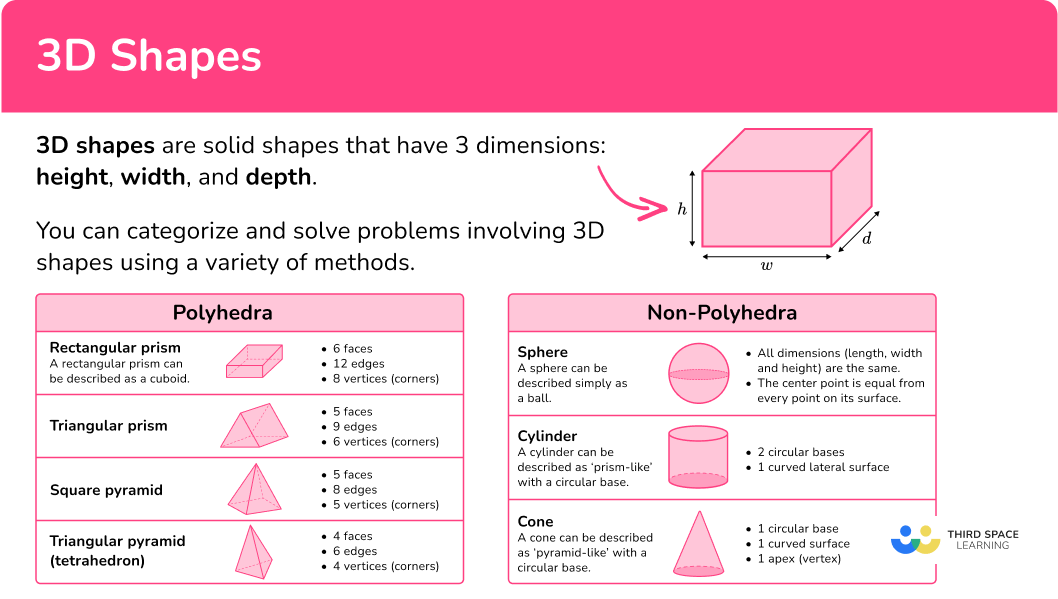## Common Core State Standards

How does this relate to 1 st grade math?

Compose two-dimensional shapes (rectangles, squares, trapezoids, triangles, half-circles, and quarter-circles) or three-dimensional shapes (cubes, right rectangular prisms, right circular cones, and right circular cylinders) to create a composite shape, and compose new shapes from the composite shape.

## How to identify 3D shapes

In order to identify a 3D shape, you will:

1. State the characteristics of the given shape.
2. Recall the definitions of the different 3D shapes and state which 3D shape the shape is.

## 3D shapes examples

### Example 1: identifying 3D shapes

What is the name of this 3D shape?

1. State the characteristics of the given shape.

The given shape has 1 circular base, 1 curved side that meets at the 1 apex on the shape.

2Recall the definitions of the different 3D shapes and state which 3D shape the shape is.

Based on the characteristics of the given shape, the shape is a cone.

### Example 2: identifying 3D shapes

Edward is giving Nancy directions to draw a given 3D shape. Edward tells Nancy that the shape has a square base with 4 triangular faces, 8 edges and 5 vertices. What shape is Edward describing to Nancy?

Edward tells Nancy that the shape has a square base with 4 triangular faces, 8 edges and 5 vertices.

Nancy recalls the shapes that she is familiar with that have a square base,

She now needs to look and see which have 4 triangular faces.

The rectangular prism and cube only have rectangular faces. These are not the shapes Edward was describing.

The triangular prism has 2 triangular faces and 3 square faces. This is not the shape Edward was describing.

The square pyramid has 1 square base and 4 triangular faces. It also has 8 edges and 5 vertices.

Edward was describing a square pyramid to Nancy.

### Example 3: real-world example

Look at the image below and determine which 3D shape it is.

The shape is a basketball, where all dimensions are the same. The center point will be equal from every point on the surface.

This shape is a sphere.

### Example 4: real-world example

Look at the image below and determine which 3D shape it is.

This shape has a square base with 4 triangular faces ( 2 showing) and has 5 vertices ( 4 showing).

This shape is a square pyramid.

## How to identify 3D shapes in a composite shape

In order to identify 3D shapes in a composite shape, you will:

1. Count the number of shapes that make up the composite shape.
2. Identify and state the number of each type of shape within the composite shape.

### Example 5: identifying 3D shapes in a composite shape

Look at the composite shape and identify all 3D shapes.

There are two shapes in this composite shape.

Shape 1 has 2 circular bases with a smooth, curved lateral face. Shape 1 is a cylinder.

Shape 2 has 6 rectangular faces that meet at vertices. Shape 2 is a rectangular prism.

### Example 6: identifying 3D shapes in a composite shape

Look at the composite shape and identify all 3D shapes.

There are \bf{7} shapes in this composite shape.

Shapes 1, 2 and 4 have 6 rectangular faces and are rectangular prisms. Shapes 1 and 2 are also classified as cubes.

Shape 3 has two rectangular faces and 3 rectangular faces. Shape 3 is a triangular prism.

Shapes 5 and 6 have 2 circular bases with a smooth, curved lateral face. These shapes are cylinders

Shape 7 has 1 circular base, 1 curved surface and 1 vertex. Shape 7 is a cone.

### Teaching tips for 3D shapes

• Find a 3D shapes song for kids to introduce and reinforce important vocabulary and concepts. Songs often contain repetitive lyrics, which reinforce the material being learned, so when students listen to or sing a song repeatedly, the information becomes ingrained in their working memory and helps recall.

• Teach terms like faces, edges, vertices, and base and incorporate these terms during discussions and activities.

• Printable worksheets that show a variety of 3D shapes or real-life examples can be useful for students to practice with. Students need to practice not being able to “see” all sides of the geometric shape, but still identify all the characteristics.

### Easy mistakes to make

• Using the wrong formula
There are several formulas for surface area and volume. Make sure you select the right one by correctly identifying the 3D shape in the question and what it is asking you to calculate.

• Using a mixture of units in a calculation
When you are calculating volume or surface area, you must ensure that the units of length you are using are the same. This may mean that you need to convert a unit before you carry out a calculation. For example, if one length is given in meters and another is given in centimeters, you must convert one of them so that they are either both in meters or both in centimeters.

• Missing or incorrect units
Surface area is measured in square units (for example, mm^{2}, cm^{2}, m^{2}, etc.).
Volume is measured in units cubed (for example, mm^{3}, cm^{3}, m^{3}, etc.).

For volume, you may also be asked to give your answer in liters or millimeters for example. Note that,
1 \, ml = 1 \, cm^{3}
1 \, liter = 1000 \, ml = 1000 \, cm^{3}

• Not counting “hidden” faces, edges and vertices
When counting the faces, edges or vertices on an image of a 3D shape, you should consider if there are any that are ‘hidden’. Some drawings use dashed lines to represent edges that cannot be seen but sometimes they do not. In this case, try and visualize if there are any ‘hidden’ faces, edges, or vertices behind or underneath the 3D representation.

### Practice 3D shapes questions

1. What is the name of this 3D shape?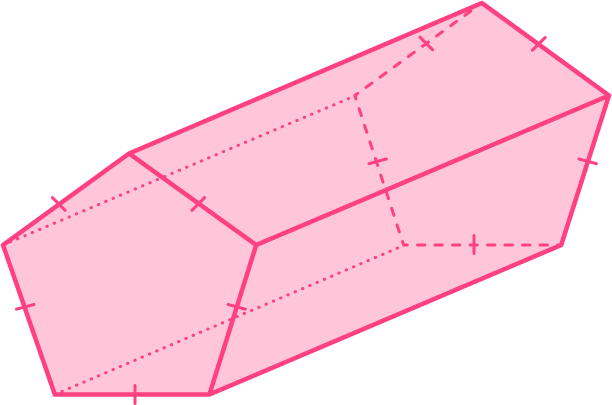Pentagonal PyramidPentagonal PrismPentagonIrregular PrismThis 3D shape has congruent cross-sectional areas, it is therefore a prism. The shape of the cross-section is a regular pentagon, so it is a pentagonal prism.

2. This is a square based pyramid. How many vertices does it have?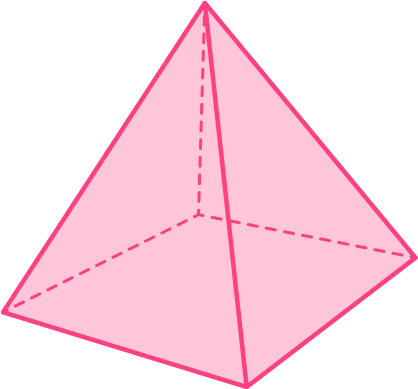4853The base of the pyramid has 4 vertices as they are the corners of a square. The apex (the highest point of the shape) is another vertex, totaling 5 .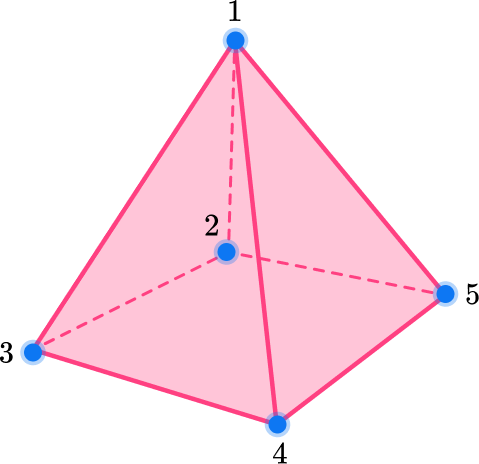3. Which shape is a triangular prism?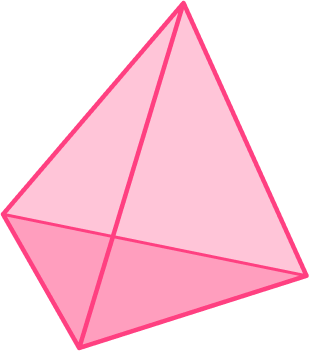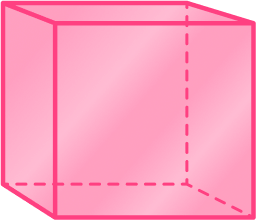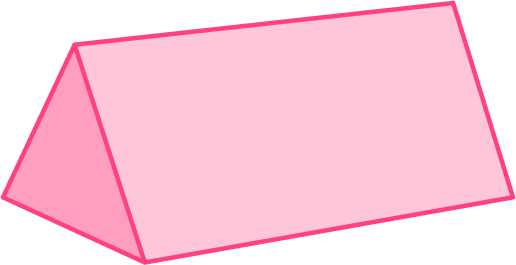A triangular prism has triangular faces, which these do not.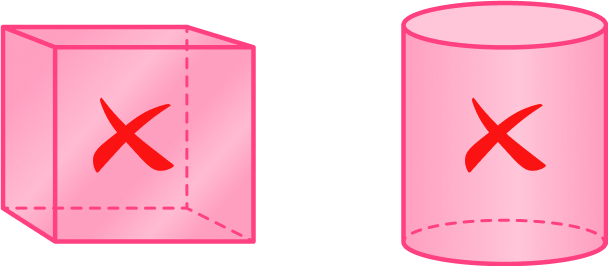A triangular prism also has three rectangular faces, which this shape does not.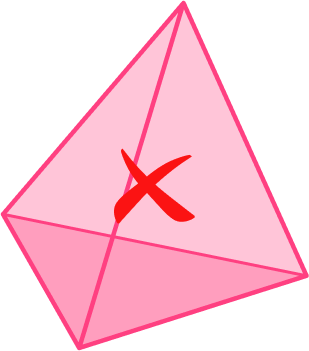This shape is a triangular prism:4. Which shape is a non-polyhedron?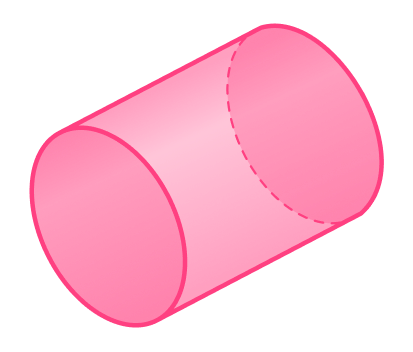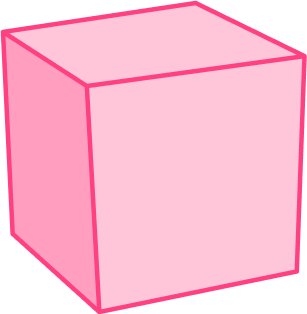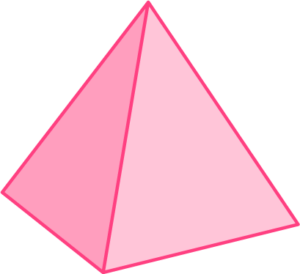Non-polyhedron shapes have at least one curved face, and these do not.This shape is a non-polyhedron: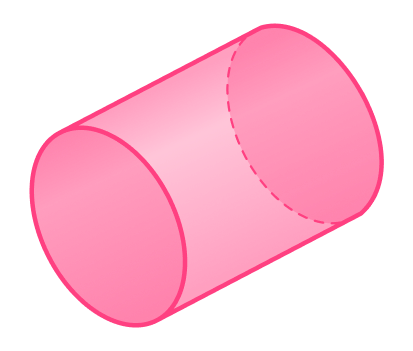5. How many cylinders are in the composite shape?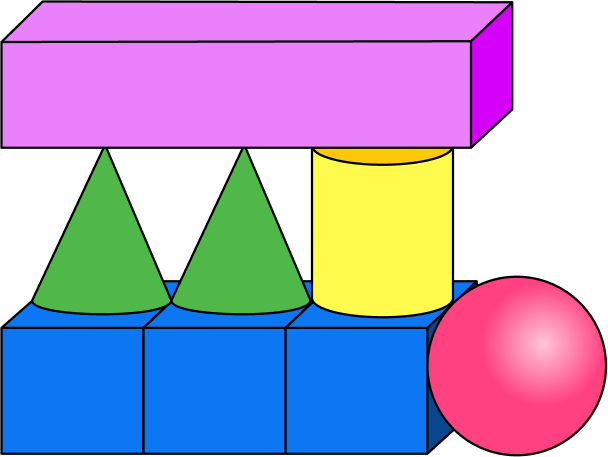3281A cylinder is a non-polydron shape with two circular bases and one curved face.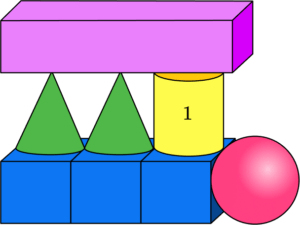There is only 1 cylinder in this composite shape.

6. Which shape is a rectangular prism?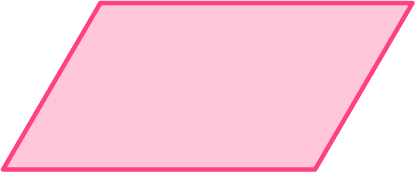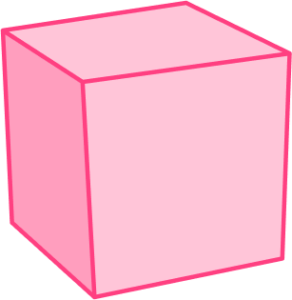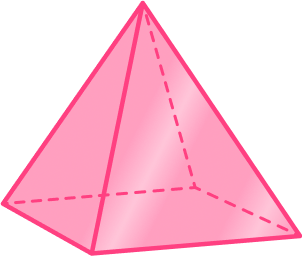A rectangular prism is a 3D shape, and a parallelogram is not 3D.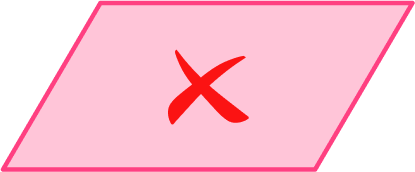A rectangular prism has 6 rectangular faces, but a cylinder has no rectangular faces and a square pyramid only has one.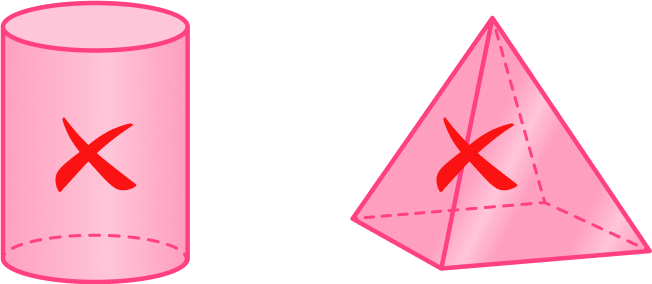This shape is a rectangular prism. It is also classified as a cube.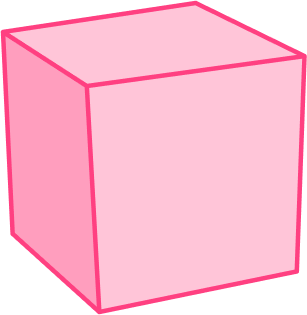## 3D shape FAQs

What is the difference between 2D and 3D shapes?

The main difference between 2D and 3D shapes lies in their dimensions. 2D shapes are flat and exist only on a plane, with length and width. They have no depth or thickness. 3D shapes have length, width, and height. They are solid, occupy space, and have a three-dimensional structure.

How do you identify a 3D shape?

To identify a 3D shape, you need to consider its properties. Look for features like faces (the flat surfaces), edges (the lines where two faces meet), and vertices (the points where edges meet). The number and arrangement of faces, edges, and vertices can help you determine the specific 3D shape.

## Still stuck?

At Third Space Learning, we specialize in helping teachers and school leaders to provide personalized math support for more of their students through high-quality, online one-on-one math tutoring delivered by subject experts.

Each week, our tutors support thousands of students who are at risk of not meeting their grade-level expectations, and help accelerate their progress and boost their confidence.